# INDIAN MATHEMATICIAN Holiday homework.pptx

30 May 2023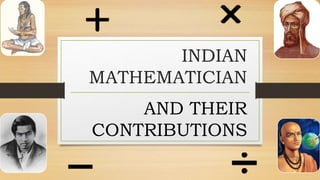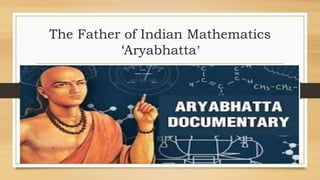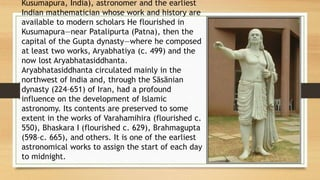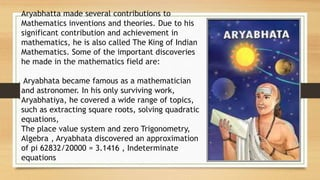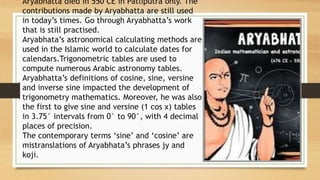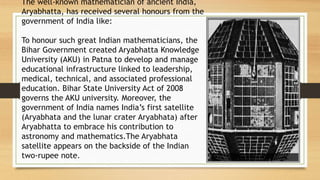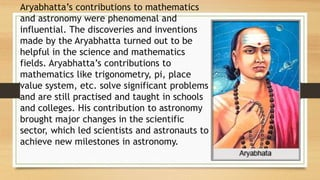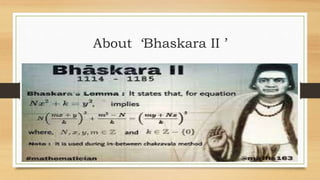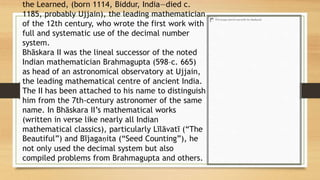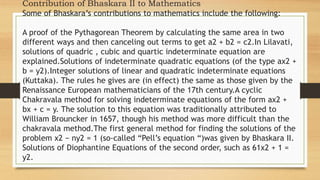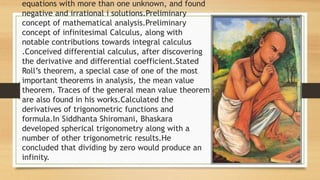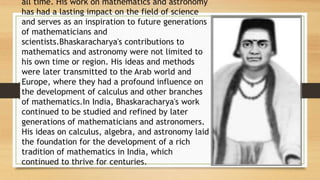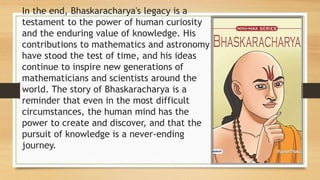1 sur 15

### INDIAN MATHEMATICIAN Holiday homework.pptx

• 2. The Father of Indian Mathematics ‘Aryabhatta’
• 3. Kusumapura, India), astronomer and the earliest Indian mathematician whose work and history are available to modern scholars He flourished in Kusumapura—near Patalipurta (Patna), then the capital of the Gupta dynasty—where he composed at least two works, Aryabhatiya (c. 499) and the now lost Aryabhatasiddhanta. Aryabhatasiddhanta circulated mainly in the northwest of India and, through the Sāsānian dynasty (224–651) of Iran, had a profound influence on the development of Islamic astronomy. Its contents are preserved to some extent in the works of Varahamihira (flourished c. 550), Bhaskara I (flourished c. 629), Brahmagupta (598–c. 665), and others. It is one of the earliest astronomical works to assign the start of each day to midnight.
• 4. Aryabhatta made several contributions to Mathematics inventions and theories. Due to his significant contribution and achievement in mathematics, he is also called The King of Indian Mathematics. Some of the important discoveries he made in the mathematics field are: Aryabhata became famous as a mathematician and astronomer. In his only surviving work, Aryabhatiya, he covered a wide range of topics, such as extracting square roots, solving quadratic equations, The place value system and zero Trigonometry, Algebra , Aryabhata discovered an approximation of pi 62832/20000 = 3.1416 , Indeterminate equations
• 5. Aryabhatta died in 550 CE in Patliputra only. The contributions made by Aryabhatta are still used in today’s times. Go through Aryabhatta’s work that is still practised. Aryabhata’s astronomical calculating methods are used in the Islamic world to calculate dates for calendars.Trigonometric tables are used to compute numerous Arabic astronomy tables. Aryabhatta’s definitions of cosine, sine, versine and inverse sine impacted the development of trigonometry mathematics. Moreover, he was also the first to give sine and versine (1 cos x) tables in 3.75° intervals from 0° to 90°, with 4 decimal places of precision. The contemporary terms ‘sine’ and ‘cosine’ are mistranslations of Aryabhata’s phrases jy and koji.
• 6. The well-known mathematician of ancient India, Aryabhatta, has received several honours from the government of India like: To honour such great Indian mathematicians, the Bihar Government created Aryabhatta Knowledge University (AKU) in Patna to develop and manage educational infrastructure linked to leadership, medical, technical, and associated professional education. Bihar State University Act of 2008 governs the AKU university. Moreover, the government of India names India’s first satellite (Aryabhata and the lunar crater Aryabhata) after Aryabhatta to embrace his contribution to astronomy and mathematics.The Aryabhata satellite appears on the backside of the Indian two-rupee note.
• 7. Aryabhatta’s contributions to mathematics and astronomy were phenomenal and influential. The discoveries and inventions made by the Aryabhatta turned out to be helpful in the science and mathematics fields. Aryabhatta’s contributions to mathematics like trigonometry, pi, place value system, etc. solve significant problems and are still practised and taught in schools and colleges. His contribution to astronomy brought major changes in the scientific sector, which led scientists and astronauts to achieve new milestones in astronomy.
• 9. the Learned, (born 1114, Biddur, India—died c. 1185, probably Ujjain), the leading mathematician of the 12th century, who wrote the first work with full and systematic use of the decimal number system. Bhāskara II was the lineal successor of the noted Indian mathematician Brahmagupta (598–c. 665) as head of an astronomical observatory at Ujjain, the leading mathematical centre of ancient India. The II has been attached to his name to distinguish him from the 7th-century astronomer of the same name. In Bhāskara II’s mathematical works (written in verse like nearly all Indian mathematical classics), particularly Līlāvatī (“The Beautiful”) and Bījagaṇita (“Seed Counting”), he not only used the decimal system but also compiled problems from Brahmagupta and others.
• 10. Contribution of Bhaskara II to Mathematics Some of Bhaskara’s contributions to mathematics include the following: A proof of the Pythagorean Theorem by calculating the same area in two different ways and then canceling out terms to get a2 + b2 = c2.In Lilavati, solutions of quadric , cubic and quartic indeterminate equation are explained.Solutions of indeterminate quadratic equations (of the type ax2 + b = y2).Integer solutions of linear and quadratic indeterminate equations (Kuttaka). The rules he gives are (in effect) the same as those given by the Renaissance European mathematicians of the 17th century.A cyclic Chakravala method for solving indeterminate equations of the form ax2 + bx + c = y. The solution to this equation was traditionally attributed to William Brouncker in 1657, though his method was more difficult than the chakravala method.The first general method for finding the solutions of the problem x2 − ny2 = 1 (so-called “Pell’s equation “)was given by Bhaskara II. Solutions of Diophantine Equations of the second order, such as 61x2 + 1 = y2.
• 11. equations with more than one unknown, and found negative and irrational i solutions.Preliminary concept of mathematical analysis.Preliminary concept of infinitesimal Calculus, along with notable contributions towards integral calculus .Conceived differential calculus, after discovering the derivative and differential coefficient.Stated Roll’s theorem, a special case of one of the most important theorems in analysis, the mean value theorem. Traces of the general mean value theorem are also found in his works.Calculated the derivatives of trigonometric functions and formula.In Siddhanta Shiromani, Bhaskara developed spherical trigonometry along with a number of other trigonometric results.He concluded that dividing by zero would produce an infinity.
• 12. all time. His work on mathematics and astronomy has had a lasting impact on the field of science and serves as an inspiration to future generations of mathematicians and scientists.Bhaskaracharya's contributions to mathematics and astronomy were not limited to his own time or region. His ideas and methods were later transmitted to the Arab world and Europe, where they had a profound influence on the development of calculus and other branches of mathematics.In India, Bhaskaracharya's work continued to be studied and refined by later generations of mathematicians and astronomers. His ideas on calculus, algebra, and astronomy laid the foundation for the development of a rich tradition of mathematics in India, which continued to thrive for centuries.
• 13. In the end, Bhaskaracharya's legacy is a testament to the power of human curiosity and the enduring value of knowledge. His contributions to mathematics and astronomy have stood the test of time, and his ideas continue to inspire new generations of mathematicians and scientists around the world. The story of Bhaskaracharya is a reminder that even in the most difficult circumstances, the human mind has the power to create and discover, and that the pursuit of knowledge is a never-ending journey.
• 15. BY CDT.RISHAV RAJ ROLL NO – 6815 HOUSE – PANDYA CLASS – IX ‘A’ SUB.TEACHER - MRS.NV